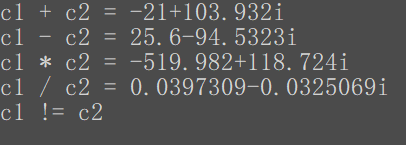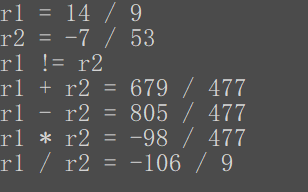• 通过构造有理数抽象数据类型，完成有理分数加减乘除和求导操作。
• 关于抽象数据类型--有理数，实现有理数的加减乘除运算
• 　数据对象：D={r,i | r,i为实数} 　数据关系：S={<r,i>} 　基本操作： 　InitComplex(&C,re,im) 　操作结果：构造一个复数C，实部为re，虚部为im。 　DestoryComplex(&C) 　初始条件：复数C...
ADT Complex{
数据对象：D={r,i | r,i为实数}
数据关系：S={<r,i>}
基本操作：
InitComplex(&C,re,im)
操作结果：构造一个复数C，实部为re，虚部为im。
DestoryComplex(&C)
初始条件：复数C存在。
操作结果：销毁复数C。
Get(C,k,&e)
初始条件：复数C存在，1<=k<=2。
操作结果：用e返回C的第k元的值。
Put(&C,k,e)
初始条件：复数C存在，1<=k<=2.
操作结果：将C的第k元改为e。
IsAscending(C)
初始条件：复数C存在。
操作结果：如果复数C的两个元素按升序排列，则返回1，否则返回0.
IsDesending(C)
初始条件：复数C存在。
操作结果：如果复数C的两个元素按降序排列，则返回1，否则返回0.
Max(C,&e)
初始条件：复数C存在。
操作结果：用e返回复数C中两个元素值较大的一个。
Min(C,&e)
初始条件：复数C存在。
操作结果：用e返回复数C中两个元素值较小的一个。
} ADT Complex

ADT RationalNumber{
数据对象：D={s,m | s,m为自然数，且m!=0}
数据关系：S={<s,m>}
基本操作：
……
}ADT RationalNumber
转载于:https://www.cnblogs.com/zhwup/p/9375588.html
展开全文• 有理数作为一个抽象数据类型 有理数 (数字在形式a/b可以被写的a和b整数，并且b不零)在计算机不能当地代表。 合理的ADT能被定义如所示下面。 建筑： 使用二个整数， a和b创造一个有理数ADT的事例， ...

有理数作为一个抽象数据类型
有理数 (数字在形式a/b可以被写的a和b整数，并且b不零)在计算机不能当地代表。 合理的ADT能被定义如所示下面。
建筑： 使用二个整数， a和b创造一个有理数ADT的事例， a代表分子，并且b代表分母。
操作： 加法，减法，增殖，分裂，取幂，比较，简化，转换向一个真正的(浮动小数点)数字。
要是一个完全规格，应该定义所有操作根据数据。 例如，当倍增二有理数a/b和c/d，结果是定义和(c)时/(b d)。 一般，输入、产品、首要条件、postconditions和假定为ADT指定。


展开全文c
• 复数、有理数C++实现
#include <iostream>
using namespace std;

class Complex {
private:
double real;
double imag;

public:
Complex(double r = 0, double i = 0);
double get_real(void);
double get_imag(void);
void set_real(double r);
void set_imag(double i);
void print(void);
friend Complex operator+(Complex &c1, Complex &c2);
friend Complex operator-(Complex &c1, Complex &c2);
friend Complex operator*(Complex &c1, Complex &c2);
friend Complex operator/(Complex &c1, Complex &c2);
friend bool operator==(Complex &c1, Complex &c2);
};

Complex::Complex(double r, double i) {
real = r;
imag = i;
}

double Complex::get_real(void) {
return real;
}

double Complex::get_imag(void) {
return imag;
}

void Complex::set_real(double r) {
real = r;
}

void Complex::set_imag(double i) {
imag = i;
}

void Complex::print(void) {
cout << real;

if (imag > 0) {
cout << "+";
}

if (imag != 0) {
cout << imag << 'i' << endl;
}
}

Complex operator+(Complex &c1, Complex &c2) {
return Complex(c1.real + c2.real, c1.imag + c2.imag);
}

Complex operator-(Complex &c1, Complex &c2) {
return Complex(c1.real - c2.real, c1.imag - c2.imag);
}

Complex operator*(Complex &c1, Complex &c2) {
return Complex(c1.real * c2.real - c1.imag * c2.imag,
c1.real * c2.imag + c1.imag * c2.real);
}

Complex operator/(Complex &c1, Complex &c2) {
double temp = 1 / (c2.real * c2.real + c2.imag * c2.imag);

return Complex((c1.real * c2.real + c1.imag * c2.imag) * temp,
(c1.imag * c2.real - c1.real * c2.imag) * temp);
}

bool operator==(Complex &c1, Complex &c2) {
return (0 == c1.real - c2.real) && (0 == c1.imag - c2.imag);
}

int main(void) {
Complex c1(2.3, 4.7), c2(-23.3, 99.2323);

cout << "c1 + c2 = " ; (c1 + c2).print();
cout << "c1 - c2 = " ; (c1 - c2).print();
cout << "c1 * c2 = " ; (c1 * c2).print();
cout << "c1 / c2 = " ; (c1 / c2).print();
c1 == c2 ? (cout << "c1 == c2" ) : (cout << "c1 != c2") << endl;

return 0;
}#include <iostream>
using namespace std;
#include <utility>

class Rational {
private:
pair<int, int> fraction;
int gcd(int x, int y) {
if (0 == x % y) {
return y;
}

return gcd(y, x % y);
}

public:
Rational(int numerator, int denominator);
pair<int, int> get_rational(void);
void set_numerator(int numerator);
void set_denominator(int denominator);
void print(void);
friend Rational operator+(Rational &r1, Rational &r2);
friend Rational operator-(Rational &r1, Rational &r2);
friend Rational operator*(Rational &r1, Rational &r2);
friend Rational operator/(Rational &r1, Rational &r2);
friend bool operator==(Rational &r1, Rational &r2);
};

Rational::Rational(int numerator, int denominator) {
if (0 != denominator) {
fraction = pair<int, int>(numerator, denominator);
} else {
fraction = pair<int, int>(1, 1);
}
}

pair<int, int> Rational::get_rational(void) {
return fraction;
}

void Rational::set_numerator(int numerator) {
fraction.first = numerator;
}

void Rational::set_denominator(int denominator) {
if (0 != denominator) {
fraction.second = denominator;
}
}

void Rational::print(void) {
if (0 == 1- fraction.second) {
cout << fraction.first << endl;
return;
}

if (0 == fraction.first) {
cout << '0' << endl;
return;
}

// 将分数最简化
int _gcd = gcd(fraction.first, fraction.second);
int a = fraction.first / _gcd;
int b = fraction.second / _gcd;

if ((a ^ b) < 0) {
a < 0 ? (cout << a << " / " << b << endl)
: (cout << -a << " / " << -b << endl) ;
return;
} else {
a > 0 ? (cout << a << " / " << b << endl)
: (cout << -a << " / " << -b << endl);
}
}

Rational operator+(Rational &r1, Rational &r2) {
return Rational(r1.fraction.first * r2.fraction.second +
r1.fraction.second * r2.fraction.first,
r1.fraction.second * r2.fraction.second);
}

Rational operator-(Rational &r1, Rational &r2) {
return Rational(r1.fraction.first * r2.fraction.second -
r1.fraction.second * r2.fraction.first,
r1.fraction.second * r2.fraction.second);
}

Rational operator*(Rational &r1, Rational &r2) {
return Rational(r1.fraction.first * r2.fraction.first,
r1.fraction.second * r2.fraction.second);
}

Rational operator/(Rational &r1, Rational &r2) {
if (0 == r2.fraction.first) {
return Rational(0, 0);
}

return Rational(r1.fraction.first * r2.fraction.second,
r1.fraction.second * r2.fraction.first);
}

bool operator==(Rational &r1, Rational &r2) {
return (0 == r1.fraction.first * r2.fraction.second -
r1.fraction.second * r2.fraction.first);
}

int main(void) {
Rational r1 = Rational(98, 63);
Rational r2 = Rational(-7, 53);

cout << "r1 = " ; r1.print();
cout << "r2 = " ; r2.print();
if (r1 == r2) {
cout << "r1 == r2" << endl;
} else {
cout << "r1 != r2" << endl;
}
cout << "r1 + r2 = " ; (r1 + r2).print();
cout << "r1 - r2 = " ; (r1 - r2).print();
cout << "r1 * r2 = " ; (r1 * r2).print();
cout << "r1 / r2 = " ; (r1 / r2).print();

return 0;
}展开全文• 实现两个有理数相加、相减、相乘以及求分子或求分母的运算。
•  有理数作为一个抽象数据类型 有理数 (数字在形式a/b可以被写的a和b整数，并且b不零)在计算机不能当地代表。 合理的ADT能被定义如所示下面。 建筑： 使用二个整数， a和b创造一个有理数ADT的事例...

有理数作为一个抽象数据类型
有理数 (数字在形式a/b可以被写的a和b整数，并且b不零)在计算机不能当地代表。 合理的ADT能被定义如所示下面。
建筑： 使用二个整数， a和b创造一个有理数ADT的事例， a代表分子，并且b代表分母。
操作： 加法，减法，增殖，分裂，取幂，比较，简化，转换向一个真正的(浮动小数点)数字。
要是一个完全规格，应该定义所有操作根据数据。 例如，当倍增二有理数a/b和c/d，结果是定义和(c)时/(b d)。 一般，输入、产品、首要条件、postconditions和假定为ADT指定。


展开全文c
• 试仿照三元组或复数的抽象数据类型写出有理数抽象数据类型的描述 （有理数是其分子、分母均为整数且分母不为零的分数）。 有理数基本运算如下所示： 1.构造有理数T，元素e1,e2分别被赋以分子、分母值 2.销毁有理数T ...
• 用ADT的抽象数据模型描述你的有理数数据类型。 ADT Rational{ 数据对象：D={e1,e2|e1,e2属于status类型} 数据关系：R1={<e1,e2>} 基本操作： Creat(&T,v1,v2) Modify(&T,i,e) A...
• 试仿照三元组或复数的抽象数据类型写出有理数抽象数据类型的描述 （有理数是其分子、分母均为整数且分母不为零的分数）。 有理数基本运算如下所示： 1.构造有理数T，元素e1,e2分别被赋以分子、分母值 2.销毁...
• 有理数抽象数据类型 2.数据结构、函数说明 头文件 main函数 3.代码实现说明 （1）构造有理数 （2）销毁有理数 （3）用e返回有理数的分子或分母 （4）改变有理数的分子或分母 （5）有理数加法 （6）有理数...
• 试仿照三元组或复数的抽象数据类型写出有理数抽象数据类型的描述 （有理数是其分子、分母均为整数且分母不为零的分数）。 有理数基本运算如下所示： 1.构造有理数T，元素e1,e2分别被赋以分子、分母值 2.销毁有理数T...
• 用ADT的抽象数据模型描述有理数数据类型 ADT Rational { 数据对象：D={e1,e2|e1,e2属于ElemType类型} 数据关系：R={<e1,e2>|e1是有理数的分子部分，e2是有理数的分母部分} 基本操作： InitRational(&T,...
• 自定义一个二元组抽象数据类型 ··· ADT Rational{ 数据对象：D={e1，e2 | e1，e2属于ElemType} 数据关系：R1={<e1，e2>} 基本操作： InitTriplet(Rational &T, ElemType v1, ElemTyp...
• 试仿照三元组或复数的抽象数据类型写出有理数抽象数据类型的描述 （有理数是其分子、分母均为整数且分母不为零的分数）。 有理数基本运算如下所示： 1.构造有理数R，元素x1,x2分别被赋以分子、分母值 2.销毁有理数R ...